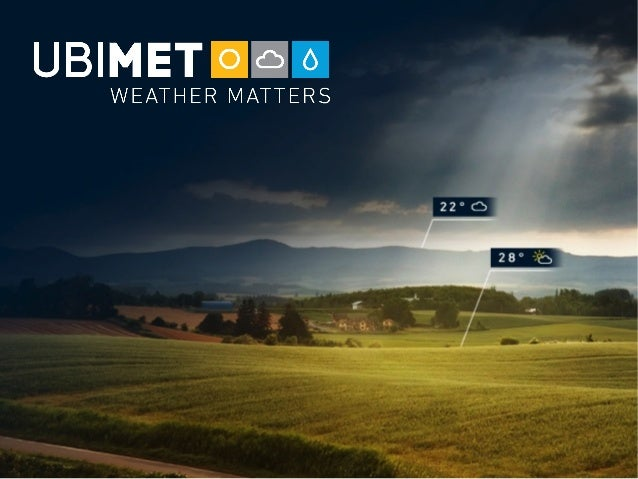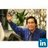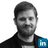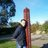Successfully reported this slideshow.Upcoming SlideShare
×

ofUpcoming SlideShare
Next

Share

# Parallel Computing With Dask - PyDays 2017

Dask is a task scheduler that seamlessly parallelizes Python functions across threads, processes, or cluster nodes. It also offers a DataFrame class (similar to Pandas) that can handle data sets larger than the available memory.

See all

See all

### Parallel Computing With Dask - PyDays 2017

1. 1. Parallel Computing With Dask Christian Aichinger
2. 2. https://greek0.net @chaichinger
6. 6. def process_cpu(url): url = url.encode() charsum = 0 for c1 in url: for c2 in url: for c3 in url: charsum += c1 * c2 * c3 return charsum [process_cpu(url) for url in urls]
7. 7. @dask.delayed def process_cpu(url): ... graph = [process_cpu(url) for url in urls] dask.compute(graph)
9. 9. @dask.delayed def f(arg): print("f", arg) return 2 * arg @dask.delayed def g(args): print("g", args) return sum(args) lst = [1, 2, 3] graph = g([f(i) for i in lst]) f-#0 g f g-#1 f-#2 f-#3 f f
10. 10. print("result", graph.compute()) f 2 f 1 f 3 g [2, 4, 6] result 12 f-#0 g f g-#1 f-#2 f-#3 f f
11. 11. Collection similar to Python lists
12. 12. import dask.bag as db db.from_sequence(urls) .map(download) .map(convert_to_image) .filter(lambda img: img.size < 500) .map(remove_artifacts) .map(save_to_disk) .compute()
13. 13. import dask.bag as db import json js = db.read_text('log-2017*.gz').map(json.loads) js.take(2) ({'name': 'Alice', 'location': {'city': 'LA', 'state': 'CA'}}, {'name': 'Bob', 'location': {'city': 'NYC', 'state': 'NY'}) result = js.pluck('name').frequencies() dict(result) {'Alice': 10000, 'Bob': 5555, 'Charlie': ...} http://dask.pydata.org/en/latest/examples/bag-json.html
14. 14. Collection similar to NumPy Arrays
15. 15. import dask.array as da import skimage.io delayed_imread = dask.delayed(skimage.io.imread, pure=True) sample = skimage.io.imread(urls) images = [delayed_imread(url) for url in urls] images = [da.from_delayed(img, dtype=sample.dtype, shape=sample.shape) for img in images] images = da.stack(images, axis=0) images.shape (1000000, 360, 500, 3)
16. 16. images.shape (1000000, 360, 500, 3) max_img = images.mean(axis=3).max(axis=0) max_img.shape (360, 500) max_img.compute() array([[ 157., 155., 153., ..., 134., 137.], [ 154., 153., 151., ..., 129., 132.], ..., [ 97., 66., 81., ..., 74., 82.]]) da.linalg.svd(max_img, 10) da.fft.fft(max_img)
17. 17. ('tensordot-#0', 2, 1, 2) sum apply ('transpose-#1', 1, 2) apply apply ('wrapped-#2', 2, 1) apply applytranspose ('tensordot-#0', 1, 1, 1) sum apply ('transpose-#1', 1, 1) apply ('wrapped-#2', 1, 1) apply transpose ('tensordot-#0', 2, 0, 2) apply ('wrapped-#2', 2, 0) apply applytranspose ('transpose-#1', 0, 2) apply apply ('tensordot-#0', 0, 1, 0) sum apply ('wrapped-#2', 0, 1) transpose ('transpose-#1', 1, 0) ('tensordot-#0', 0, 0, 2) sum ('wrapped-#2', 0, 0) apply apply transpose ('tensordot-#0', 2, 0, 0) sum ('transpose-#1', 0, 0) apply ('tensordot-#0', 0, 0, 0) ('tensordot-#0', 2, 2, 0) apply ('wrapped-#2', 2, 2) apply applytranspose ('transpose-#1', 2, 0) apply apply ('tensordot-#0', 0, 2, 2) apply ('transpose-#1', 2, 2) apply ('wrapped-#2', 0, 2) apply transpose ('tensordot-#0', 1, 0, 1) apply ('transpose-#1', 0, 1) ('wrapped-#2', 1, 0) transpose ('tensordot-#0', 2, 1, 0) ('tensordot-#0', 0, 2, 1) sum ('transpose-#1', 2, 1) apply ('tensordot-#0', 0, 2, 0) ('tensordot-#0', 0, 0, 1) ('tensordot-#0', 0, 1, 2) ('tensordot-#0', 1, 2, 1) ('wrapped-#2', 1, 2) transpose ('tensordot-#0', 2, 2, 2) ('tensordot-#0', 1, 2, 2) sum ('tensordot-#0', 2, 2, 1) sum ('tensordot-#0', 1, 0, 0) sum ('tensordot-#0', 1, 1, 0)('tensordot-#0', 2, 0, 1) ('tensordot-#0', 0, 1, 1)('tensordot-#0', 1, 2, 0) ('tensordot-#0', 1, 0, 2) ('tensordot-#0', 2, 1, 1) ('tensordot-#0', 1, 1, 2)('sum-#3', 2, 0) ('sum-#3', 0, 0) ('sum-#3', 0, 1) ('sum-#3', 2, 2) ('sum-#3', 1, 2)('sum-#3', 0, 2) ('sum-#3', 1, 1)('sum-#3', 2, 1) ('sum-#3', 1, 0) onesones onesones onesones ones ones ones ('tensordot-#0', 2, 1, 2) sum apply ('transpose-#1', 1, 2) apply ('wrapped-#2', 2, 1) appltranspose ('tensordot-#0', 2, 0, 2) apply ('wrapped-#2', 2, 0) applytranspose ('transpose-#1', 0, 2) apply ('tensordot-#0', 0, 0, 2) sum ('wrapped-#2', 0, 0) apply transpose ('tensordot-#0', 2, 0, 0) sum ('transpose-#1', 0, 0) ('tensordot-#0', 0, 0, 0) ('tensordot-#0', 2, 2, 0) apply ('wrapped-#2', 2, 2) apply transpose ('transpos ('tensordot-#0', 0, 2, 2) apply ('transpose-#1', 2, 2) ('wrapped-#2', 0, 2 trans ('tensordot-#0', 2, 1, 0) ('tensordot-#0', 0, 1, 2)('tensordot-#0', 2, 2, 2) ('sum-#3', 2, 0) ('sum-#3', 2, 2) ('sum-#3', 0, 2) ones ones onesones ones
18. 18. Collection similar to Pandas Dataframes
19. 19. __Request received (wms) : #17236, 2016-12-27 16:03:44.898007, current_connections = connected=4, accepted=4, idle threads=4 appid="mapcache" client_ip=10.0.39.1 user_agent="..." query=… __Request processed (wms) : #17236, total_duration=00:00:11.377182 cache_hits=7917 cache_misses=0 success_rate=100% successes=262144 failures=0
20. 20. RE_REQ_RECEIVE = re.compile(r""" __Request receiveds+ ((?P<iface>w+))s*:s* # Interface (wfs, wms) #(?P<req_id>d+),s* # Request id (?P<starttime>[^,]+),s* # Request start timestamp current_connections=s* ... """, re.VERBOSE) RE_REQ_PROCESSED = re.compile(r""" __Request processeds+ (w+)s*:s* # Interface (wfs, wms) #(?P<req_id>d+),s* # Request id total_duration=(?P<total_duration>[0-9:.]+)s+ ... """, re.VERBOSE)
21. 21. bag = db.read_text(files) ddf_recv = (bag .str.strip() .map(lambda line: REQ_RECEIVE.match(line)) .remove(lambda el: el is None) .map(lambda m: m.groupdict()) .to_dataframe(columns=pd.DataFrame(columns=RECV_COLS)) ) ddf_proc = (bag ...) requests = ddf_recv.merge(ddf_proc, on='req_id', how='inner')
22. 22. slow_req = requests[ (requests.starttime >= datetime(2017, 5, 1) & (requests.starttime < datetime(2017, 5, 2) & (requests.total_duration >= timedelta(seconds=5))] slow_req = slow_req.compute(get=dask.multiprocessing.get)
24. 24. from distributed import Client client = Client('10.0.0.8:8786')
25. 25. Image Credit ● UBIMET background and company logo Used with permission ● CPU frequency scaling: Created by Wikipedia user Newhorizons msk, in the public domain https://en.wikipedia.org/wiki/File:Clock_CPU_Scaling.jpg ● Parallel computing: Created by the US government, in the public domain https://computing.llnl.gov/tutorials/parallel_comp/ ● Python logo: A trademark of the Python Software Foundation https://www.python.org/community/logos/ ● Dask logo: Part of the Dask source distribution, licensed BSD v3 https://github.com/dask/dask/blob/master/docs/source/images/dask_horizontal.svg ● All charts and graphs: created myself ● Bag By Pixabay user “OpenClipart-Vectors”, in the public domain https://pixabay.com/p-156023/?no_redirect ● Array Jerome S. Higgins, in the public domain https://commons.wikimedia.org/wiki/File:Land_Act_of_1785_section_numbering.png ● Frame Modified form of a Wellcome Trust image, licensed CC-BY 4.0 https://commons.wikimedia.org/wiki/File:Picture_frame_Wellcome_L0051764.jpg ● Dask Array Composition of NumPy Arrays, Dask DataFrame Composition of Pandas Dataframes Partially modified, part of the Dask source distribution, licensed BSD v3 All from https://github.com/dask/dask/blob/master/docs/source/images/ ● Cluster: Created by Julian Herzog, licensed GNU FDL v2 / CC-BY 4.0 https://commons.wikimedia.org/wiki/File:High_Performance_Computing_Center_Stuttgart_HLRS_2015_08_Cray_XC40_Hazel_Hen_IO.jpg ● Dask Distributed graph: Partially modified, part of the Dask source distribution, licensed BSD v3 https://github.com/dask/dask/blob/9f344bbf38610e03f723ac034f9c4a390a7debec/docs/source/images/distributed-layout.svg
•#### mikepham12

Nov. 8, 2018
•#### GrPatassy

Aug. 26, 2018
•#### EduardoRL2

Apr. 14, 2018

Total views

1,365

On Slideshare

0

From embeds

0

Number of embeds

1

28

Shares

0